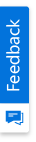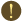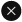We use cookies to give you the best experience on our website. If you continue to browse, then you agree to our privacy policy and cookie policy.Unfortunately, activation email could not send to your email. Please try again.# ASP.NET MVC Bubble Chart - Visualize & Compare the Relationships

## Overview

ASP.NET MVC Bubble Chart is like scatter chart, but allows you to visualize data in 3 dimensions. The size of the bubble is determined based on the third parameter.

## Customizing each point in bubble

Appearance of each data point in a bubble chart can be customized using point render event to differentiate each point based on the size.

## Data label

Data labels display information about data points. Add a template to display data labels with HTML elements such as images, DIV, and spans for more informative data labels. You can rotate a data label by its given angle.

## ASP.NET MVC Bubble Chart Code Example

Easily get started with ASP.NET MVC Bubble Chart using a few simple lines of C# code example as demonstrated below. Also explore our ASP.NET MVC Bubble Chart Example that shows you how to render and configure the chart.

```@Html.EJS().Chart("container").Series(series =>
{
}).PrimaryXAxis(px => px.ValueType(Syncfusion.EJ2.Charts.ValueType.Category)).Render();```
```public class HomeController : Controller
{
public ActionResult Index()
{
List<ChartData> chartData = new List<ChartData>
{
new ChartData{ x=2000, y1= 0.03, y2= 0.48},
new ChartData{ x=2001, y1= 0.05, y2= 0.53 },
new ChartData{ x=2002, y1= 0.06, y2= 0.57 },
new ChartData{ x=2003, y1= 0.09, y2= 0.61 },
new ChartData{ x=2004, y1= 0.14, y2= 0.63 },
new ChartData{ x=2005, y1= 0.20, y2= 0.64 },
new ChartData{ x=2006, y1= 0.29, y2= 0.66 },
new ChartData{ x=2007, y1= 0.46, y2= 0.76 },
new ChartData{ x=2008, y1= 0.64, y2= 0.77 },
new ChartData{ x=2009,  y1= 0.75, y2= 0.55 }
};
ViewBag.dataSource = chartData;
return View();
}
}
public class ChartData
{
public double x;
public double y1;
public double y2;
}```

## Learning ResourcesBubble Chart User Guide

Learn the available options to customize ASP.NET MVC Bubble Chart.Bubble Chart API Reference

Explore the ASP.NET MVC Bubble Chart APIs.

### 80+ ASP.NET MVC UI CONTROLS

ALL CONTROLS
VIEWERYou are using an outdated version of Internet Explorer that may not display all features of this and other websites. Upgrade to Internet Explorer 8 or newer for a better experience.